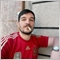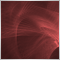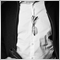# Simple question2745

Hi there community, I've got a doubt on this lines.

```   double GlobalTarget=(((Positive+PositionsTotal())/2)*Equity)/100;
double TakeProfit=GlobalTarget;if(Positive>0){
TakeProfit=NormalizeDouble(GlobalTarget/Positive,2);
GlobalTarget=GlobalTarget/(PositionsTotal()/Positive);}```

Once the condition becomes true (Positive>0), the GlobalTarget used to get the TakeProfit value would be the first one (above) or the last one (below)?7253

David Diez:

Hi there community, I've got a doubt on this lines.

Once the condition becomes true (Positive>0), the GlobalTarget used to get the TakeProfit value would be the first one (above) or the last one (below)?

you will find things easier to understand if you lay your code out better..

once the IF statement becomes true then the code between the { } will execute and change the values accordingly

```double GlobalTarget=(((Positive+PositionsTotal())/2)*Equity)/100;
double TakeProfit=GlobalTarget;
if(Positive>0)
{
TakeProfit=NormalizeDouble(GlobalTarget/Positive,2);
GlobalTarget=GlobalTarget/(PositionsTotal()/Positive);
}```2082

Somehow the first line is disturbing.

PositionsTotal returns the amount of open positions. How is this related to equity and why is the result a double?

What could be the sense of dividing the product by 2?

And even if this were for a "position basket" why equity? Do all positions have the same lot size? Isn't that assumption a little vague?

This doesn't feel right.2745

Huh, I've deleted this post as I've got no answers along the day and I found that the way I've coded is correct. The TakeProfit is taken from the GlobalTarget above.

Paul Anscombe #:

you will find things easier to understand if you lay your code out better..

once the IF statement becomes true then the code between the { } will execute and change the values accordingly

Your code is the same as I wrote. You're wrong from the point that the lines above are what they count as far as I could see.

Dominik Christian Egert #:
Somehow the first line is disturbing.

PositionsTotal returns the amount of open positions. How is this related to equity and why is the result a double?

What could be the sense of dividing the product by 2?

And even if this were for a "position basket" why equity? Do all positions have the same lot size? Isn't that assumption a little vague?

This doesn't feel right.

Quite simple, the GlobalTarget needs to be defined based on equity and positionstotal(). How else?

When you divide a sum by an integer you get an average.

I prefer using equity instead of balance, lotsize is according to tickvalue and atr. What's doesn't feel right?

Everything is f*****g perfect.7253

David Diez #:

Huh, I've deleted this post as I've got no answers along the day and I found that the way I've coded is correct. The TakeProfit is taken from the GlobalTarget above.

Your code is the same as I wrote. You're wrong from the point that the lines above are what they count as far as I could see.

Quite simple, the GlobalTarget needs to be defined based on equity and positionstotal(). How else?

When you divide a sum by an integer you get an average.

I prefer using equity instead of balance, lotsize is according to tickvalue and atr. What's doesn't feel right?

Everything is f*****g perfect.

You did get answers you just don’t like them
How can I be wrong when I correctly answered your question of what gets processed according to the if statement as you asked !
Seems to me you need learn the basics2745

Paul Anscombe #:
You did get answers you just don’t like them
How can I be wrong when I correctly answered your question of what gets processed according to the if statement as you asked !
Seems to me you need learn the basics

Don't be annoying.

```double GlobalTarget=(((Positive+PositionsTotal())/2)*Equity)/100;
double TakeProfit=GlobalTarget;if(Positive>0){
TakeProfit=NormalizeDouble(GlobalTarget/Positive,2); // At this point GlobalTarget is the line above.
GlobalTarget=GlobalTarget/(PositionsTotal()/Positive);} // This line becomes the new GlobalTarget value using the first value.```27511

David Diez #: Don't be annoying.

Like you were at 388657#comment_27652887, 370848#comment_22737037, 322656#comment_13288449, and 318861/page2#comment_12618331? After three (3) years you still haven't changed.2745

William Roeder #:

Like you were at 388657#comment_27652887, 370848#comment_22737037, 322656#comment_13288449, and 318861/page2#comment_12618331? After three (3) years you still haven't changed.

My apologies, there are days better than others.

Still I think I am right thus there's no need to argue on this matter.